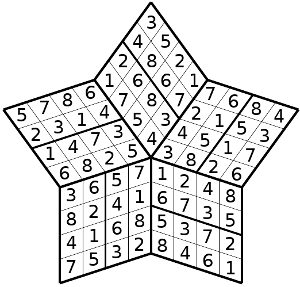Rules of Spark Sudoku

## Rules of Spark Sudoku

I am proud of this Sudoku variant and I believe that I am the first to offer you this kind of puzzle. This puzzle with its variety of form and size will please everyone.

The Spark Sudoku is a sudoku in a shape of star. It has two kinds of puzzle: the Spark where each point contains 16 cells and the Super Spark Sudoku where the points has 36 cells. Like as Sudoku, the goal is to place the number from 1 to 8 in each region, row and column (for the Super Spark it is the number 1 to 12). The particularity is that each row and column spread on two points of the star.They are 4 variants of Spark Sudoku:

• Star with 5 points: pentagram.
• Star with 6 points: hexagram.
• Star with 7 points: heptagram.
• Star with 8 points : octogram.

This is an example of pentagram  (5 points)Spark Sudoku Pentagram                                  Spark Sudoku Solution

Notice that each row and column occupy two points and each point has two regions.

Now the hexagram (6 points)

The puzzle

spark_4_6_00001.pdf

spark_4_6_00001_sol.pdf

The heptagram (7 points)

The puzzle

spark_4_7_00001.pdf

spark_4_7_00001_sol.pdf

And finally the octogram  (8 points).

The puzzle

spark_4_8_00001.pdf

spark_4_8_00001_sol.pdf

### Super Spark Sudoku

The Super Spark Sudoku is different from the Spark Sudoku by its size, each point has 3 regions and you have to fill the number 1 to 12 in each region,row and column. It appears in 4 forms of star ( 5 to 8 points) .

So, this is a pentagram,

The puzzle

spark_6_5_00001.pdf

spark_6_5_00001_sol.pdf

and a heptagram (7 points).

The puzzle

spark_6_7_00001.pdf

spark_6_7_00001_sol.pdf

Don't hesitate to take the challenge by resolving these puzzles.